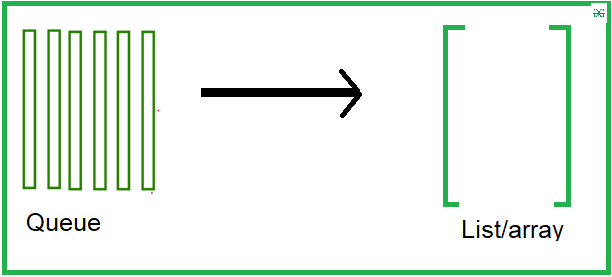# Dumping queue into list or array in Python

Prerequisite: Queue in Python

Here given a queue and our task is to dump the queue into list or array. We are to see two methods to achieve the objective of our solution.Example 1:

In this example, we will create a queue using the collection package and then cast it into the list

## Python3

 `# Python program to ` `# demonstrate queue implementation ` `# using collections.dequeue ` `  `  `from` `collections ``import` `deque ` `  `  `# Initializing a queue ` `q ``=` `deque() ` `  `  `# Adding elements to a queue ` `q.append(``'a'``) ` `q.append(``'b'``) ` `q.append(``'c'``) ` ` `  `# display the queue ` `print``(``"Initial queue"``) ` `print``(q,``"\n"``) ` ` `  `# display the type ` `print``(``type``(q)) `

Output:

```Initial queue
deque(['a', 'b', 'c'])

<class 'collections.deque'>```

Let’s create a list and cast into it:

## Python3

 `# convert into list ` `li ``=` `list``(q) ` ` `  `# display ` `print``(``"Convert into the list"``) ` `print``(li) ` `print``(``type``(li)) `

Output:

```Convert into the list
['a', 'b', 'c']
<class 'list'>```

Example 2:

In this example, we will create a queue using the queue module and then cast it into the list.

## Python3

 `from` `queue ``import` `Queue ` ` `  `# Initializing a queue ` `que ``=` `Queue() ` ` `  `# Adding elements to a queue ` `que.put(``1``) ` `que.put(``2``) ` `que.put(``3``) ` `que.put(``4``) ` `que.put(``5``) ` ` `  `# display the queue ` `print``(``"Initial queue"``) ` `print``(que.queue) ` ` `  `# casting into the list ` `li ``=` `list``(que.queue) ` `print``(``"\nConverted into the list"``) ` `print``(li)`

Output:

```Initial queue
deque([1, 2, 3, 4, 5])

Converted into the list
[1, 2, 3, 4, 5]```

Feeling lost in the world of random DSA topics, wasting time without progress? It's time for a change! Join our DSA course, where we'll guide you on an exciting journey to master DSA efficiently and on schedule.
Ready to dive in? Explore our Free Demo Content and join our DSA course, trusted by over 100,000 geeks!